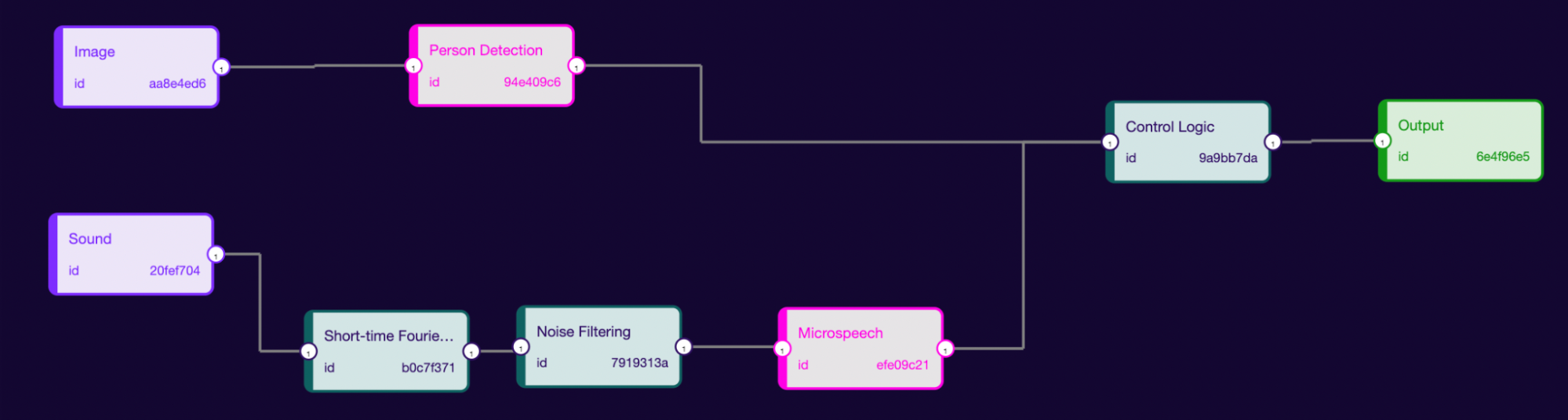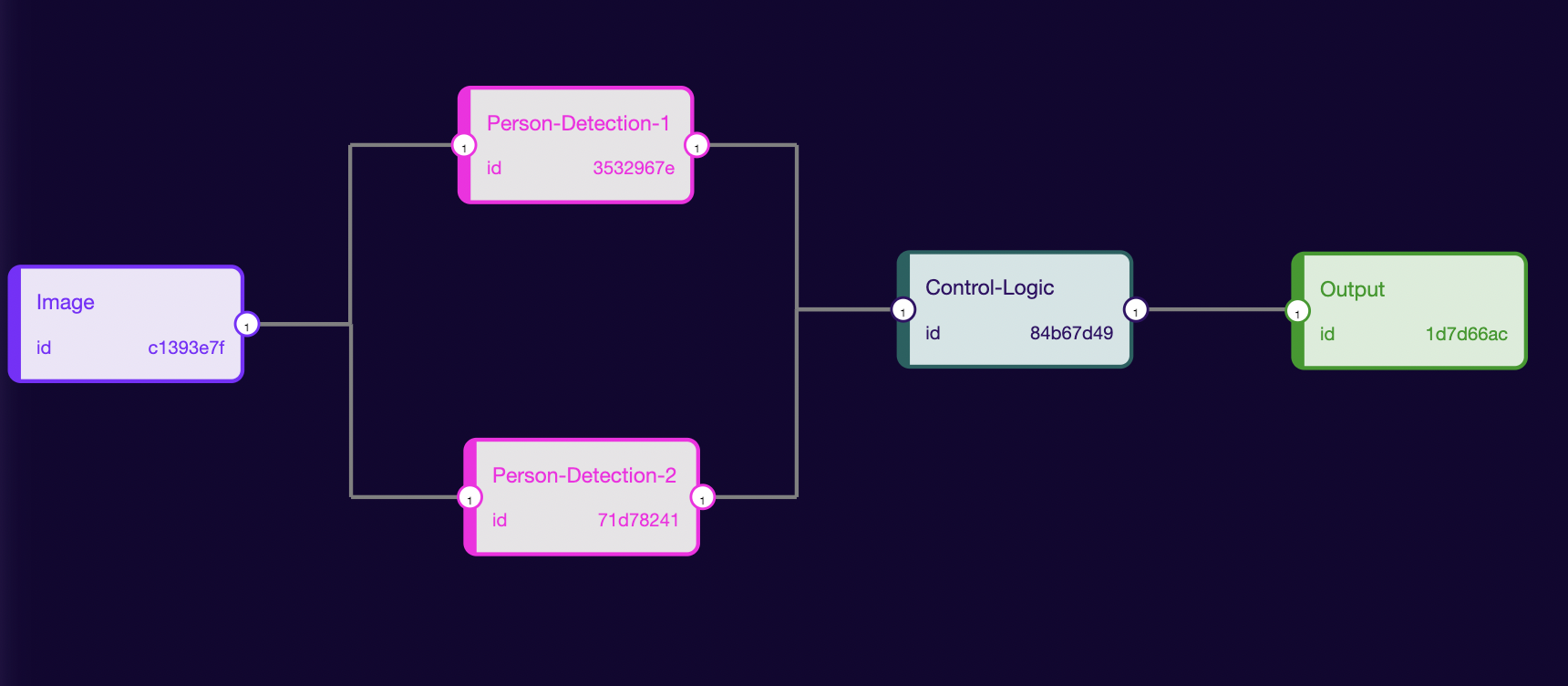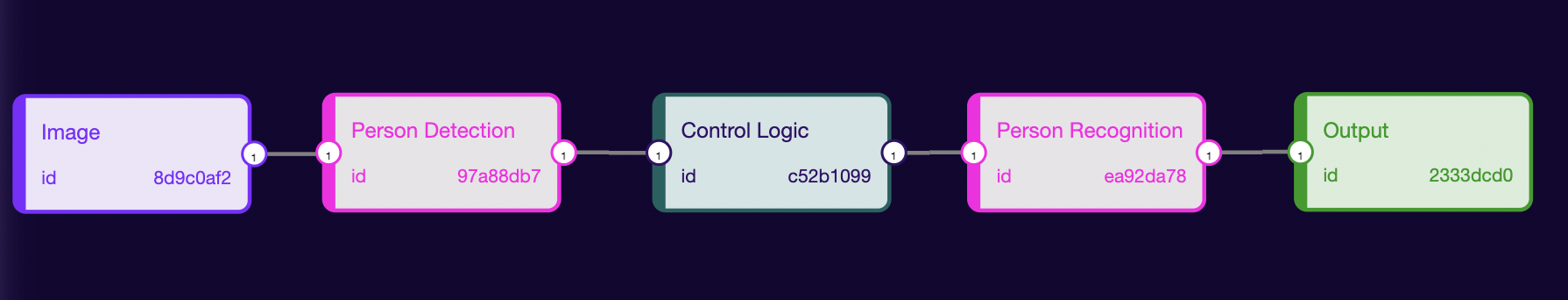# Controlling Nodes

## 1. Parallel Connections​

There are two different inputs source. Each for a different model.In the above pipeline, we are taking two inputs (Image and Sound). If there would be a person in the Image then only we will output the MicroSpeech model predictions.

How this could be useful

There could be two parallel models and we may want to output the result of the second model based on the output of the first model.It’s possible to run two different models for the same task parallelly and output the prediction of one with a high confidence value. Like in the above image, we will use two different person detection models parallelly.

It’s hard to do such kinds of things with any other technology but could be achieved with the rune. This is just a glimpse of what could be achieved.Person Detection: An image classification model takes an image as input and detects whether a person is present in the image.

Person Recognition: An image detection model which will draw bounding boxes and segment maps over the person.

In this pipeline, we are first detecting whether there is a person in the image. If there would be a person then only we will pass the image matrix to the second model.

How this could be Useful

Let’s say we have a very high computation-powered model. We will split the model into two models.

1. Low computation powered model
2. High computation powered model

The first model could be a lightweight model which requires very little computation power for its inference. If the first model detects a person then only we will run inference on the second model which requires very high computation power. It will result in:

• Low Power Consumption: We will only be running a very low-powered model. The second high computation-powered model will only run when required.

• Inference Time Reduction: The second model will not run inference for the unwanted images. There may be times when we will skip some unwanted inputs and will only run our second model on the useful data.

• Latency Reduction: We have reduced the amount of computation required to run inference using a model, resulting in lower latency.